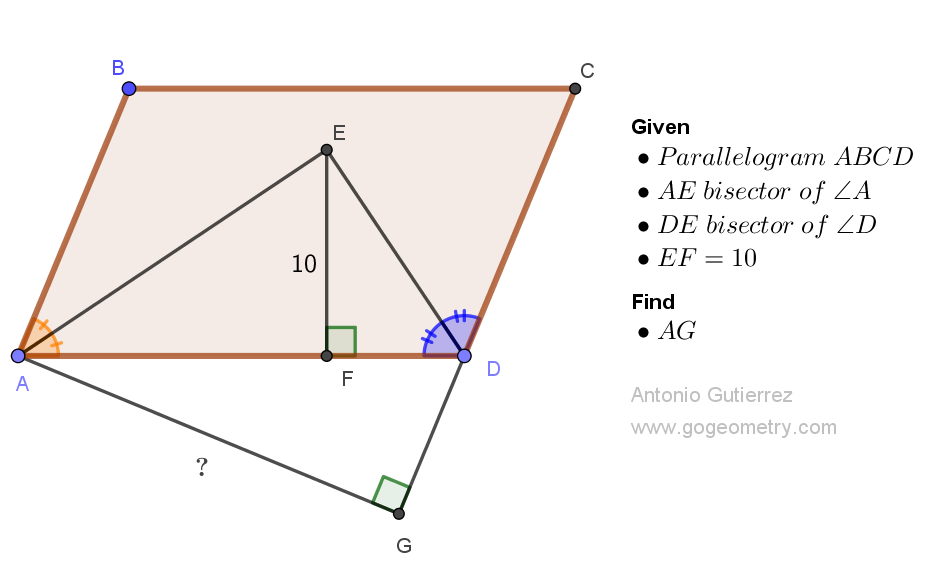# Geometry Problem 1526: Mastering Geometry Problem-Solving: Discover the Distance Between Two Sides in a Parallelogram Using Bisectors and Distance Measures. Difficulty Level: High School.

Given parallelogram ABCD with bisectors of angles A and D intersecting at point E, and the distance from E to AD measuring 10 units, determine the distance from A to CD in units.## Geometry Tips Now!

Concept Definition Theorem / Comment
Triangle A polygon with three sides and three angles. The sum of the angles in a triangle is 180 degrees.
Parallelogram A parallelogram is a quadrilateral with both pairs of opposite sides parallel. Opposite sides of a parallelogram are congruent; opposite angles are congruent; consecutive angles are supplementary; diagonals bisect each other.
Angle bisector A line or ray that divides an angle into two congruent angles.. The Angle Bisector Equidistant Theorem states that if a point lies on the angle bisector of an angle, then it is equidistant from the two sides of the angle.
Parallel lines Two lines in a plane that do not intersect. If two parallel lines are cut by a transversal, then the alternate interior angles are congruent, the corresponding angles are congruent, and the consecutive interior angles are supplementary.
Distance The distance between two geometric figures is generally defined as the minimum distance between any two points, one on each of the two figures..
Perpendicular lines Two lines or line segments that intersect at a right angle. Two lines perpendicular to a same line are parallel to each other.
Rectangle A rectangle is a two-dimensional shape with four straight sides and four right angles (90-degree angles). In a rectangle, opposite sides are congruent and parallel.
Congruence Two triangles are said to be congruent if all corresponding sides and angles of one triangle are equal to the corresponding sides and angles of the other triangle.. There are several ways to prove that two triangles are congruent, including the Side-Side-Side (SSS), Side-Angle-Side (SAS), Angle-Side-Angle (ASA), and Hypotenuse-Leg (HL) criteria.
Auxiliary line Auxiliary line is a line that is added to a diagram in order to help prove a theorem or solve a problem. Often, an auxiliary line is drawn to create additional congruent or similar triangles, to create parallel lines, or to create right angles. The use of auxiliary lines can simplify a problem or make a proof more straightforward. However, it is important to ensure that the auxiliary line does not create any new intersections or angles that were not present in the original diagram.

### Thematic Poem: Solve the Parallelogram Challenge with Bisectors and Distances

Amidst the lines and angles that we see,
A challenge awaits, a geometry mystery,
Mastering problem-solving, the key,
To unlock the secrets of symmetry.

A parallelogram, ABCD its name,
Bisectors and distances, the tools to claim,
Find the distance from A to CD,
With precision and skill, solve it with glee.

A journey of discovery, a quest to prevail,
With logic and reasoning, let us unveil,
The distance between two sides we seek,
A problem to solve, a victory to keep.

So take on the challenge, with courage and might,
As we journey together, towards problem-solving light,